# 9 Best Discrete Math Classes Online with Learning Material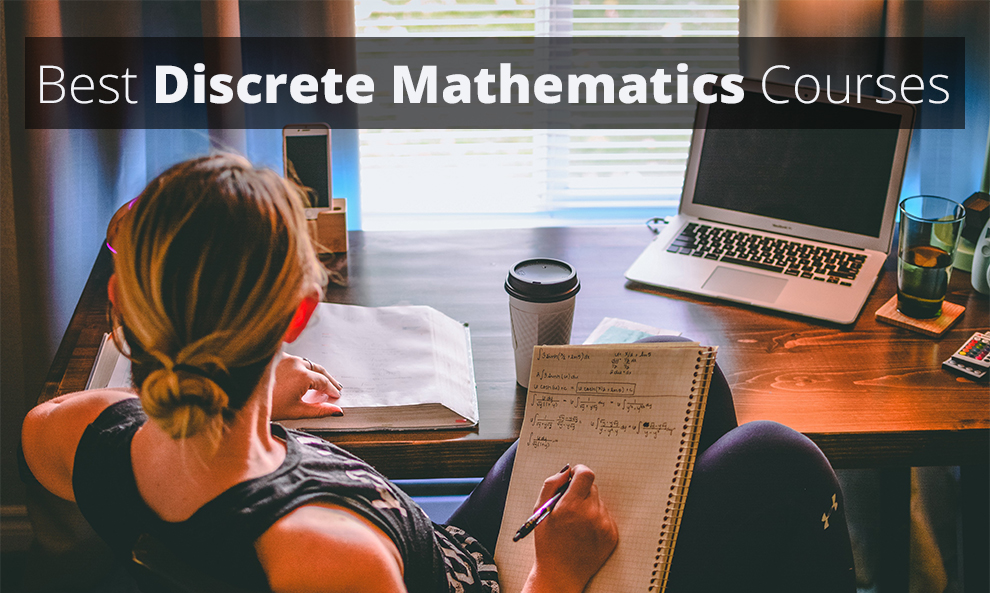Discrete math or Discrete Mathematics (DM) is the backbone of computer science and mathematics. In a DM course, you will cover the topics which are discrete and not continuous, and every CS or Math Student should necessarily take up a course in DM.

The topics included in a discrete math online course are the ones that touch every Science or Math student at some point or other in their education. Choosing a good discrete mathematics online course will equip you well and develop a solid foundation for concepts.

Some concepts that you will learn in discrete math are:

• Relational and database theory
• Algorithms
• Data structures
• Linear and abstract algebra
• Logic
• Combinatorics
• Probability
• Set theory
• Number theory, etc.

Overall, this branch of mathematics provides the students with the ability to comprehend mathematics better. Thus, if it is an essential aspect of your learning, you must enroll in a course to learn discrete math online. This guide covers nine of the best discrete math courses. Let us get started and address these courses one by one.

## 9 Best Discrete Mathematics Online Courses

### 1. Discrete Mathematics – [Udemy]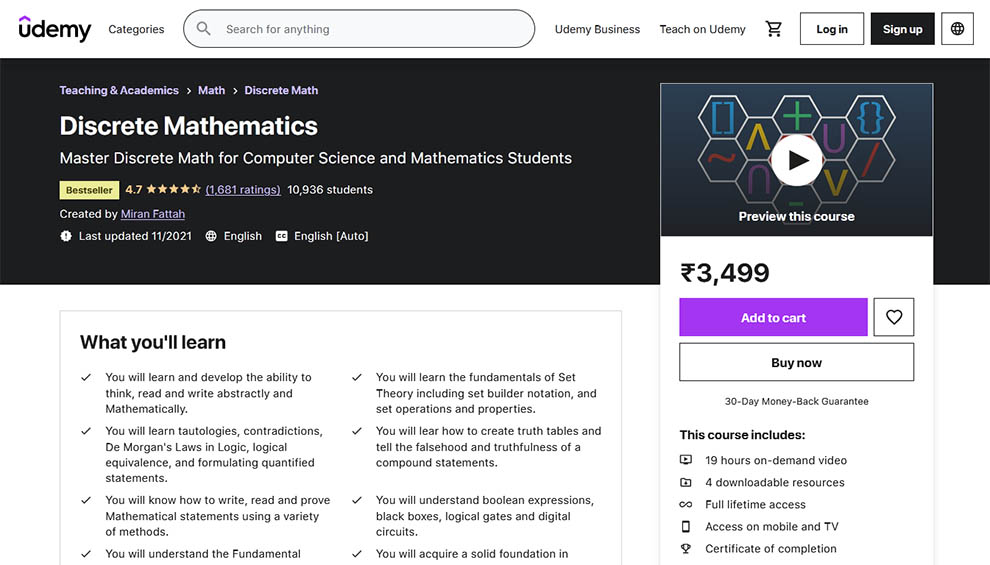Rating 4.7 Enrolled 10,807 students Duration 19 hours on-demand video Certification Yes Paid Yes Instructor Miran Fattah Return or refund policy 30-day money-back guarantee Cons The pace of the instructor is quite fast. This can be challenging for the learners.

Right at the top of the list is this Udemy bestseller, and indeed the best online course for discrete mathematics. It has an array of quizzes, which can help you ascertain how well you have understood the course.

Across the course, the instructor has delivered the content most simplistically and straightforwardly. You will learn via intuitive videos, making even the most complex content easy to understand.

Everything is taught in simple English, and no complicated mathematical jargon is included in the course. More so, whatever resources are included in the course come with lifetime access. There is a Q&A section in the course. So, you can clear your doubts quickly.

The course is divided into the following sections:

• Sets
• Logic
• Number Theory
• Proofs
• Functions
• Relations
• Graph Theory
• Statistics
• Combinatorics
• and Sequences and Series

#### Learning Outcomes

In this discrete math class online, you will learn:

• The ability to think and write with statistical and mathematical understanding.
• Fundamentals of Set theory, including set operations, set builder notation, and properties
• Tautologies, contradictions, De Morgan’s Laws in Logic, logical equivalence, and formulating quantified statements
• How to create the truth tables
• About the falsehood and truthfulness of compound statements
• The right way for writing, reading, and proving the mathematical statements with an array of different methods.
• What are black boxes, boolean expressions, digital circuits, and logical gates
• Vitalities around the Fundamental Theorem of Arithmetic’s, modular arithmetic, and learning how to find GCD & LCM
• The functions, function composition & combination, bijective, and inverse functions
• How to find the equivalence classes and equivalence relations
• Essential concepts in Statistics and Combinatorics
• About the partial sums and geometric and arithmetic sequences
• Fundamental concepts of the graph theory, including the adjacency and incidence matrices, walks, eccentricity, Hamiltonian paths and circuits, connectedness, and Ore’s Theorem.

#### Prerequisites

To learn discrete math online with this course, you must have a fair background in algebra.

#### Who should take this course?

This best discrete mathematics online course is suitable for:

• Students majoring or minoring in Computer Science, Engineering, Math, or Mathematics Education

Reviews by Armen:

This is a great course covering a lot of topics. You can definitely start your Discrete Math journey with this course.

### 2. Master Discrete Mathematics: Sets, Math Logic, and More – [Udemy]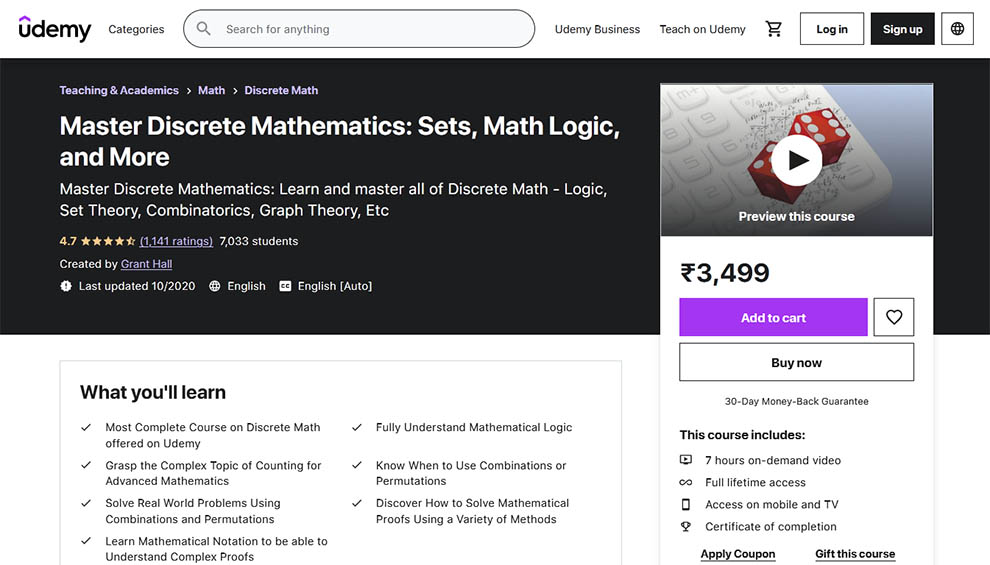Rating 4.5 Enrolled 7,014 students Duration 7 hours on-demand video Certification Yes Paid Yes Instructor Grant Hall Return or refund policy 30-day money-back guarantee Cons Though it is broadcasted as a comprehensive course, it is not enough.

This is an exquisite course designed to help you master the complicated topics in discrete math to prepare you for a career in actuarial science, computer science, engineering, or mathematics.

The course has more than 30 lectures, introducing you to different topics, such as sets and their properties. In addition, you will also learn some advanced counting techniques via the course.

Across this best online course for discrete mathematics, your progress will be assessed via the different quizzes and videos, with examples covering just about every topic.

#### Learning Outcomes

There are many things to learn in this best discrete math online course. These include:

• A thorough understanding of the mathematical logic
• The complex topic of counting for advanced mathematics
• Solving real-world problems with permutations and combinations
• Learning the mathematical notation to understand complex proofs
• Learning to solve the mathematical proofs with an array of different methods

#### Prerequisites

The vital prerequisites to take this discrete math class online are:

• Willingness to learn
• Time and patience
• Fundamentals of math

#### Who should take this course?

This course is ideal for:

• Anyone who wishes to expand their mathematical knowledge
• People wanting to learn the math logic
• People who want to know the math behind computers
• Math majors
• Computer science majors

Reviews by Anshuman Borkakoti:

Quick course covering the basics of discrete mathematics in a crisp and well-explained manner. I really liked the chalkboard illustrations.

### 3. Master Discrete Math 2020: More Than 5 Complete Courses In 1 – [Udemy]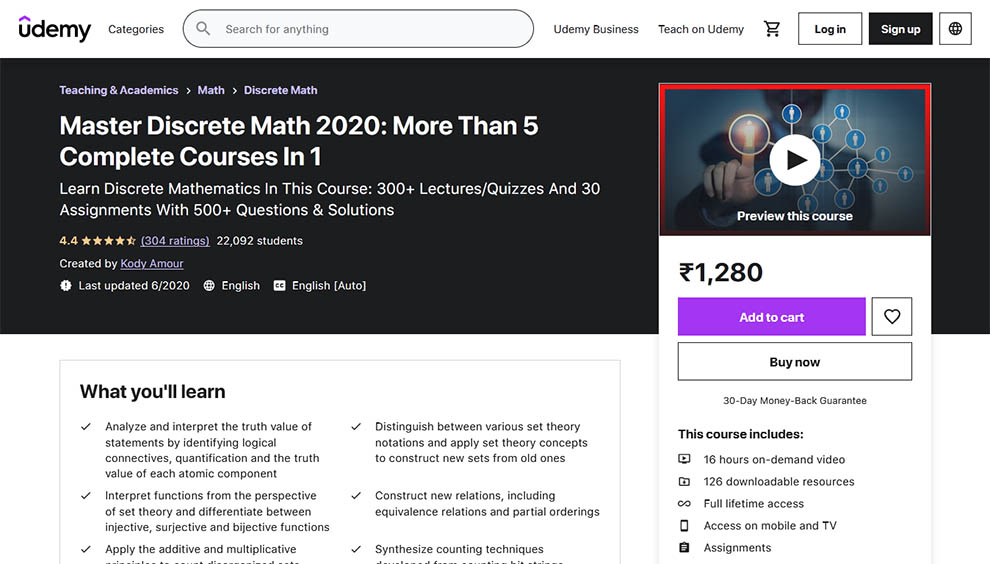Rating 4.4 Enrolled 22,077 students Duration 16 hours on-demand video Certification Yes Paid Yes Instructor 22,077 students Return or refund policy 30-day money-back guarantee Cons In several parts of the course, the presentation is quite poor.

If you wish to take just one course to learn discrete math online, this can be the best pick. This is a 461-lesson course, which has a plethora of text and video explanations, which cover everything you ought to know about discrete math.

There are 150 quizzes in the course and their solutions. Every lecture is designed to assess your understanding of what you have learned. In addition, there are 30 workbooks and over 500 additional practice questions with solutions to test what you have learned.

Overall, it is a comprehensive and straightforward course for discrete math.

This course is divided into twenty-four sections. These include:

• Mathematical Statements
• Set Theory
• Functions And Function Notation
• Relations
• Binomial Coefficients
• Combinations And Permutations
• Combinatorial Proofs
• Advanced Counting Using The Principle Of Inclusion And Exclusion
• Describing Sequences
• Arithmetic And Geometric Sequences
• Polynomial Fitting
• Solving Recurrence Relations
• Mathematical Induction
• Propositional Logic
• Proofs And Proving Techniques
• Graph Theory Definitions
• Trees
• Planar Graphs
• Coloring Graphs
• Euler Paths And Circuits
• Matching In Bipartite Graphs
• Generating Functions
• Number Theory

#### Learning Outcomes

Some things you will learn in this discrete math class online are:

• Interpreting and analyzing the true value of the statements by identifying the truth value of each atomic component, logical connectives, and quantification.
• Understanding the difference between different set theory notations
• Interpreting the functions from the point of view of set theory
• Applying the set theory concepts to construct new sets from old ones
• Learning the difference between surjective, injective, and bijective functions
• Proving the formulas with complex counting techniques involving bars and stars
• Applying the multiplicative and additive principles to count disorganized sets
• Constructing new relations, such as partial orderings and equivalence relations
• Synthesizing counting techniques from binomial coefficients, lattice paths, and counting bit strings
• Proving some formulas are true with special combinatorial proofs.
• Formulating the counting techniques for approaching challenging problems with permutations and combinations
• Drafting closed formulas and recurrence relations for several sequences.
• Connecting between counting functions and complex counting problems with certain properties
• Knowledge of the different types of graphs using coloring schemes
• Applying different proving techniques, such as proof by contradiction, proof by contrapositive, and direct proofs to prove different mathematical statements
• Analyzing different graphs with definitions from graph theory
• Learning the properties of planar graphs with Euler’s Formula
• Employing the generating functions to solve sophisticated recurrence relations
• Forming a deep understanding of the number theory that involve patterns in the natural numbers
• Applying the concepts from graph theory, such as matching problems and properties of bipartite graphs
• Creating different properties of Hamiltonian paths and cycles and Euler paths and circuits
• Solving several recurrence relations with polynomial fitting
• Discovering the several algorithms and properties involved in graph theory
• Understanding the properties and the relationships involving geometric and arithmetic sequences
• Using truth tables and Boolean Algebra to find the true value of complex molecular statements
• Mastering the strong induction and mathematical induction to prove sophisticated statements including natural numbers by solving an array of examples
•

#### Prerequisites

To take this discrete math class online, you need:

• Preparing yourself for some complex content
• Preparation to succeed in any college-level course
• Readiness to learn some excellent stuff
• Knowledge of high school algebra

#### Who should take this course?

This best discrete mathematics online course is suitable for:

• Anyone who aspires to learn about Discrete math, irrespective of their prior experience
• Beginners with no prior knowledge or understanding of discrete math
• Students who have a decent knowledge of discrete math but wish to upgrade their knowledge

Reviews by Gabor Somogyvari:

Clear explanations, and clear examples. I understand the guy 100%, and thats all I need for a good course. I am halfway though and I am happy I chose this course in particular.

### 4. Introduction to Discrete Mathematics for Computer Science Specialization – Offered by University Of California San Diego HSE University – [Coursera]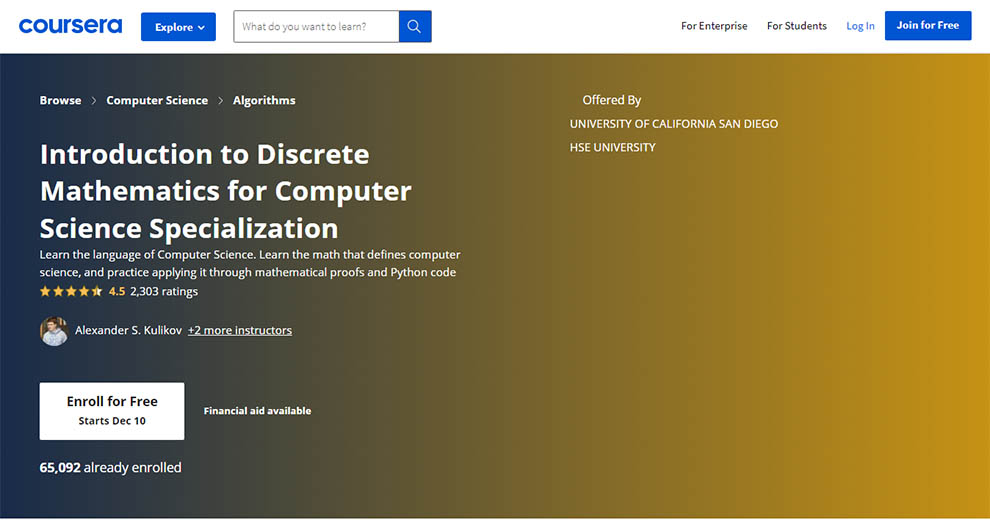Offered by University Of California San Diego HSE University Rating 4.5 Enrolled 64,786 students Duration Approximately 6 months to complete Suggested pace of 5 hours/week Certification Yes Paid Yes Instructor Alexander S. Kulikov, Vladimir V. Podolskii, and Michael Levin Return or refund policy 7-days trial Cons Some puzzles are not in sync with the lessons in the course.

In this course, to learn discrete math online, you will understand this complex language in a fun and engaging manner. Across the course, you will solve several interactive puzzles exquisitely designed to help you assess your understanding of the concepts learned in this specialization. There are a total of five courses in this specialization.

#### Who should take this course?

This is a beginner-level discrete math class online. So, even someone with no prior experience will appreciate the course.

#### Prerequisites

As such, no prior information or knowledge is required for you to take this course.

### 5. Discrete Mathematics – Offered by Shanghai Jiao Tong University – [Coursera]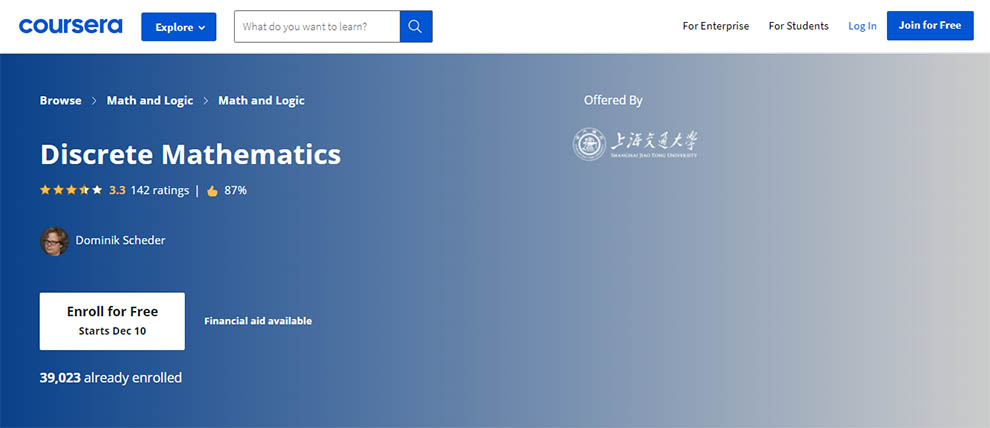Offered by Shanghai Jiao Tong University Rating 3.3 Enrolled 38,913 students Duration Approx. 42 hours to complete Certification Yes Paid Yes Instructor Dominik Scheder Return or refund policy 14-day money-back guarantee Cons There lacks some clarity in certain aspects of the course.

It is a rigorous course, sans any formality. It implies that every concept in the course will cover at least one interesting and one not-so-interesting result, along with its proof. The good thing is it is all done sans any formal notation, employing figures and examples, as and when possible.

#### Learning Outcomes

There are multiple things to learn in this discrete mathematics online course. A few of them are:

• Familiarity with different math objects, such as graphs, functions, sets, and relations, which are widely used in computer science
• Understanding of the formal statements and their proofs
• Coming up with the rigorous proof yourself
• Learning about the enumerative combinatorics
• Understanding of the network flow and matchings and the graph theory

However, you will not study modular arithmetic, algebra, and logic in this course, as these topics have a different flavor. You can find other courses on Coursera for the same.

#### Prerequisites

This is an intermediate-level discrete math online course. So, to take the course, you must have familiarity with college-level or school-level algebra.

Reviews by IA:

This course requires some background knowledge of mathematics, and the exercises require some hard work. It’s interesting, engaging, and has a lot of knowledge on offer!

## Bonus Discrete Math Class Online

### 1. Discrete Mathematics for Computer Science – [Harvard University]This is a great course to learn discrete math online, which teaches you all the math components, which are not taught in the traditional linear algebra or calculus sequences, which is mandatory to take some advanced courses in the theory of algorithms or computation.

So, the course will teach you discrete mathematics, basic probability, and logic but does not teach linear or calculus algebra.

Further, the course will also introduce you to writing formal proofs and reading mathematical notation. The prime objective of the course is to teach a set of mathematical topics and prepare you to think mathematically and read and write mathematics.

This is an instructor-led course, and you will have specific hours to take the course.

#### Prerequisites

This is an advanced-level course. So, you must have some basic information about the subject before you enroll in the course.

### 2. Discrete Mathematics Online College Course – [University of North Dakota]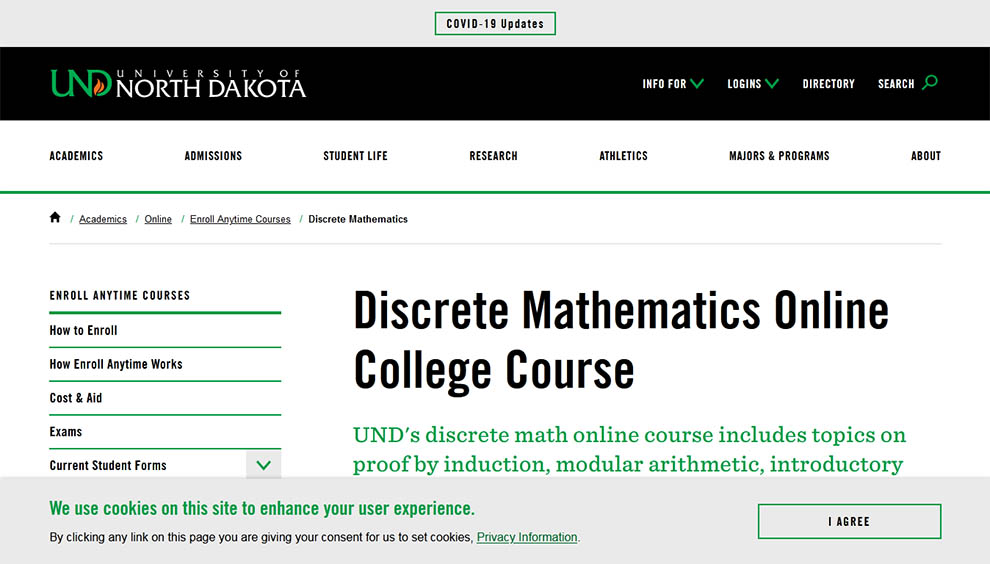Next, we have another self-paced course by the University of North Dakota. It can be enrolled at any time and will take anywhere between three to nine months for completion. When you complete this discrete math class online, you will earn three credits.

#### Prerequisites

For this discrete math online course, you will need:

MATH103: College Algebra or MATH107: Precalculus or Math Placement Exam Score

#### Learning Outcomes

If you take this course to learn discrete math online, you will know about the following:

• Symbolic logic
• Set theory
• Relations
• Functions
• Proofs by induction
• Recurrence relations
• Counting techniques

### 3. Discrete Mathematics Courses – [edX]If you need the best discrete math class online, edX can be your go-to platform. They have several discrete mathematics online courses that can simplify the learning process. You can indeed find the best course that fits your budget, needs, experience, duration, and skill level. Browse through the courses, and make your selection.

### 4. Programming Foundations: Discrete Mathematics – [LinkedIn Learning]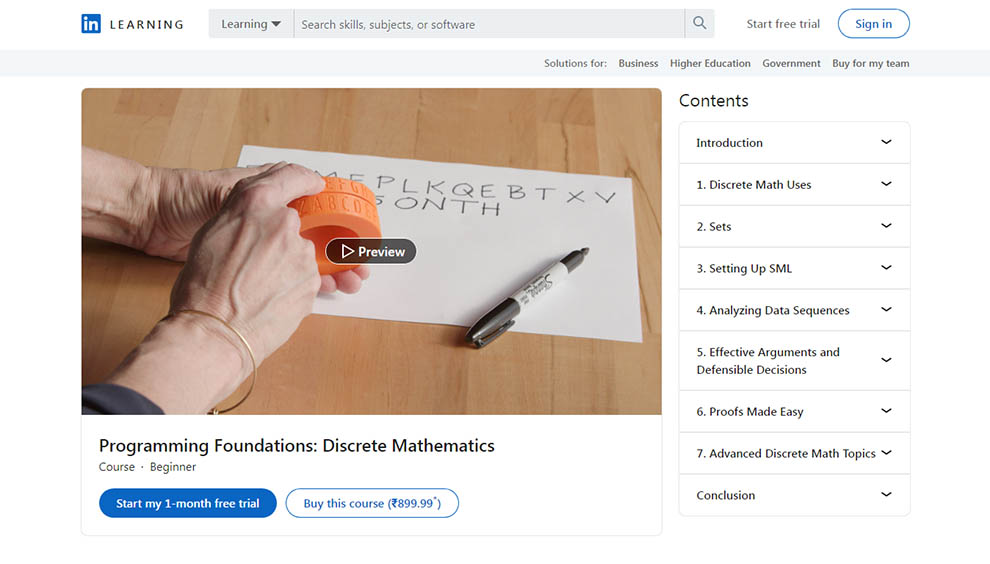This course by LinkedIn Learning or Lynda depends on the open-source SML library to demonstrate discrete math concepts. Your instructor for this course is Peggy Fisher. It will take around three hours for you to complete this course and learn discrete math online.

#### Learning Outcomes

In this course you will understand:

• Manipulating the data sets
• Writing truth tables and proofs
• Analyzing the data sequences
• Visualizing data with graph theory

#### Prerequisites

This is a beginner-level course. So, no prior knowledge on the subject is needed.

## Conclusion

So, these are the top nine best online courses for discrete mathematics. We have tried to include all the relevant information about these best discrete math online courses in this guide.

If you aspire to know more about any of the above-listed discrete math classes online, you can click on the link above and find details. Some vital details include the reviews on the course, instructor bio, knowledge and experience, enrolment date, and the course fee.

So, go ahead, find the requisite details, and make your selection. Regardless of your choice, it will be an excellent discrete math class online.Best Discrete Math Classes Online Reviewed by 10 Discrete Math Experts 4.6